Test: Dimensional Geometry - 9

# Test: Dimensional Geometry - 9

Test Description

## 20 Questions MCQ Test Topic-wise Tests & Solved Examples for IIT JAM Mathematics | Test: Dimensional Geometry - 9

Test: Dimensional Geometry - 9 for Mathematics 2023 is part of Topic-wise Tests & Solved Examples for IIT JAM Mathematics preparation. The Test: Dimensional Geometry - 9 questions and answers have been prepared according to the Mathematics exam syllabus.The Test: Dimensional Geometry - 9 MCQs are made for Mathematics 2023 Exam. Find important definitions, questions, notes, meanings, examples, exercises, MCQs and online tests for Test: Dimensional Geometry - 9 below.
Solutions of Test: Dimensional Geometry - 9 questions in English are available as part of our Topic-wise Tests & Solved Examples for IIT JAM Mathematics for Mathematics & Test: Dimensional Geometry - 9 solutions in Hindi for Topic-wise Tests & Solved Examples for IIT JAM Mathematics course. Download more important topics, notes, lectures and mock test series for Mathematics Exam by signing up for free. Attempt Test: Dimensional Geometry - 9 | 20 questions in 60 minutes | Mock test for Mathematics preparation | Free important questions MCQ to study Topic-wise Tests & Solved Examples for IIT JAM Mathematics for Mathematics Exam | Download free PDF with solutions
 1 Crore+ students have signed up on EduRev. Have you?
Test: Dimensional Geometry - 9 - Question 1

### The equation of one of the tangents to the circle x2+y2 -6x+4y= 12 parallel to tlie straight line 4x+3y+5 = 0, is given by

Detailed Solution for Test: Dimensional Geometry - 9 - Question 1

Follow the two steps given below
1. Any straight line parallel to
4x + 3y + 5 = 0
will be
4x + 3y + c = 0
2. If (i) touches the given circle then they intersect at one point only.

Test: Dimensional Geometry - 9 - Question 2

### The equation of the normal at point (x', y') of the circle x2+ y2+ 2gx + 2fy + c = 0 is given by

Detailed Solution for Test: Dimensional Geometry - 9 - Question 2

The given circle is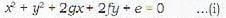Normal at the point,(x', y') is a line passing through it and perpendicular to the tangent at the point.
∴Its equation is given by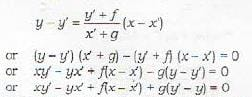Test: Dimensional Geometry - 9 - Question 3

### From any given point outside the circle, how many tangents can be drawn to a circle?

Detailed Solution for Test: Dimensional Geometry - 9 - Question 3

Remark: Let p be the given point. Then
(i) Exactly two tangents to the circle can be drawn if p is an outside point.
(ii) Only one tangent to the circle can be drawn if the point p is on the circle.
(iii) No tangents (or imaginary' tangents) to the circle can be drawn if P is inside the circle.

Test: Dimensional Geometry - 9 - Question 4

xx1 + yy1 = a2 is the equation of the

Detailed Solution for Test: Dimensional Geometry - 9 - Question 4

Remark : 1. The following three equations coincide:
(i) Equation of the tangent to a circle at a point P(x1, y1)
(ii) Equation of the chord of contact of the tangents to the circle from an outside point (x1, y1)
(iii) Equation of the polar of a point (x,  y) inside or outside the circle (but not on the circle), with repsect to the circle.
Remark: 2. If the point P(x1, y1) is on the circle, then its polar coincides with the tangent at that point.
Remark: 3. If the polar of a point P passes through a point Q, then the polar of Q passes through P.

Test: Dimensional Geometry - 9 - Question 5

The pole of a given line Ax + By + C = 0 with respect to the circle x2 + y2 = a2, is given by

Test: Dimensional Geometry - 9 - Question 6

The pole of the straight line 9x + y = 28 with 2x2 + 2y2 -3x + 5x - 7 = 0 is the point whose coordinates are

Test: Dimensional Geometry - 9 - Question 7

Which of ihr following statements is incorrect

Test: Dimensional Geometry - 9 - Question 8

The coordinates of the point, from which the lengths of the tangents to the circles
x2+ y2- 16x + 60 = 0
3x2 + 3y2 - 36x +81=0
x2 + y2- 16x- 12y + 84 = 0
are equal, are given by

Test: Dimensional Geometry - 9 - Question 9

The length of the tangent drawn from the point (6 , - 7) to the circle 3x2 + 3y2 - 7x - 6y-12 = 0 is given by

Test: Dimensional Geometry - 9 - Question 10

If (R, α) is the centre of the circle in terms of polar coordinates and a be its radius, then the polar equation of such circle is given by

Detailed Solution for Test: Dimensional Geometry - 9 - Question 10

Let (r, θ) be a point on the circle.
Then the distance between (r, θ) and (R, α) = a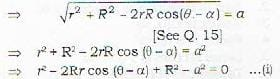Test: Dimensional Geometry - 9 - Question 11

Which of the following is/are the condition(s) that the line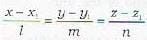may lie in the plane ax + by - cz + d = 0, if

Detailed Solution for Test: Dimensional Geometry - 9 - Question 11

Condition for a line to lie in a plane.
Let the line be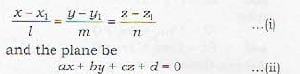Then the line will lie in the plane if every point on the line lies in the plane.
Taking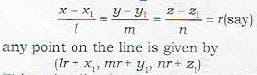This point lies in plane (ii), if it satisfies the equation of the plane.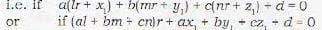This equation will be true for every value of r^i.e. every point of line (1) will lie in the plane), if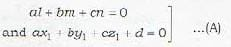Thus conditions in (A) are the required conditions.

Test: Dimensional Geometry - 9 - Question 12

Which of the following lines lies in the plane x + 2y - 3z = 6?

Detailed Solution for Test: Dimensional Geometry - 9 - Question 12

Notts that
(a) Satisfies both the conditions in (A)
(b) S a tisfies first in (A) and not the second.
(c) .Also satisfies first in (A) and not the second.
(d) .Also satisfies first, in (A) and not the second It. means that the three straight lines in (b), (c) and (d) are perpendicular lo the normal to the plane. In ot her words, these three straight lines are parallel to the plane but do not lie in the point c.

Test: Dimensional Geometry - 9 - Question 13

The equation of the plane passing through the point (x1, y1, z1) and through the line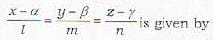Detailed Solution for Test: Dimensional Geometry - 9 - Question 13

To find the equation of a plane passing through a given point (x1, y1, z1) and a given line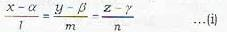The equation of the plane through the line (i) is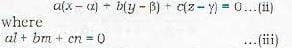Since the plane (ii) passes through the point (x1, y1, z1), therefore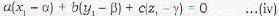Eliminating a, b, c from equations (ii), (iii) and (iv), we get the required equation of the plane as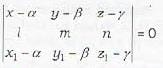Test: Dimensional Geometry - 9 - Question 14

The equation of the plane containing the lines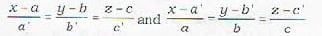is not given by

Detailed Solution for Test: Dimensional Geometry - 9 - Question 14

To find the equation of the plane containing the lines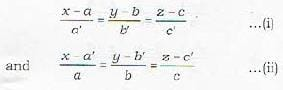The plant: containing (i) is
A ( x - a) + B ( y - b) + C ( z - c) = 0 ...(iii)
where Aa' + Bb‘ + Cc' 3 =0 ...(iv)
Further, since the plane (iii) also contains line (ii),  therefore , the normal to plane (iii) is perpendicular to line (ii).
∴ Aa + Bb + Cc = 0 ...(v)
Eliminating A, B, C from equations (iii), (iv) and (v), we get the required equation of plane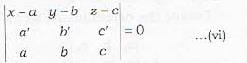This is statement (b). The statements (a) and (c) can be deduced from this by simple propert ies of determinants. Thus (a), (b|, (c) are correct.

Test: Dimensional Geometry - 9 - Question 15

How many arbitrary constants occur in the equation of a straight line in general?

Detailed Solution for Test: Dimensional Geometry - 9 - Question 15

The number of arbitrary constants in the equation of a straight line in general.
We know that a given line can be regarded as the intersection of any two planes. In particular, we may take- the two planes perpendicular to two of the coordinate planes, say yz and zx planes. These planes are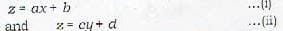Equations (i) and (ii) thus represent the given line. Note that equations (i) and (ii) contain four arbitrary constant a, b, c, d

Test: Dimensional Geometry - 9 - Question 16

The equation of the perpendicular drawn from the point (2, 4, -1) to the line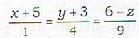are given b

Detailed Solution for Test: Dimensional Geometry - 9 - Question 16

Given point is : (2, 4, -1)
Given line is :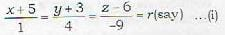The coordinates of any points on line (i) are
(r - 5, 4r - 3, -9r + 6)
This will be the required foot of perpendicular if the line joining it to the point (2, 4. -1) is perpendicular to the given line.
This requires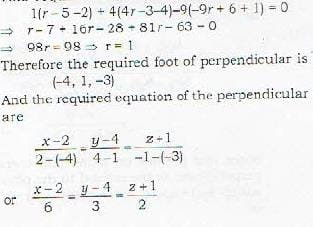Test: Dimensional Geometry - 9 - Question 17

What is the magnitude of the line of shortest distance between the lines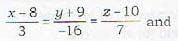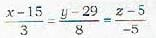Detailed Solution for Test: Dimensional Geometry - 9 - Question 17

Magnitude and equations of Shortest Distance (S.D)
Here we have to find the magnitude and the equation of shorttest distance between two given lines.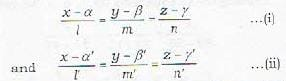*    It
By definition, the line of shortest distance between (i) and (ii) is perpendicular to both (i) and (ii). Therefore, if [λ,μ,ν] an; the d.c.’s of the line of shortest distance we have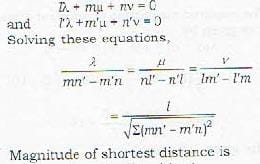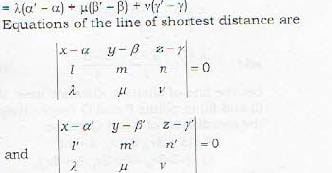Remark: Two lines given by (i) and (ii) will intersect, i.e. these lines will be coplanar if shortest distance between them is zero. Thus the condition for two lines to be coplanar is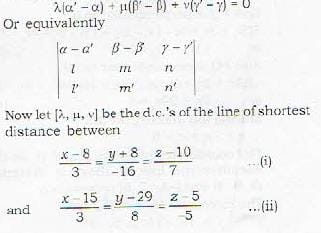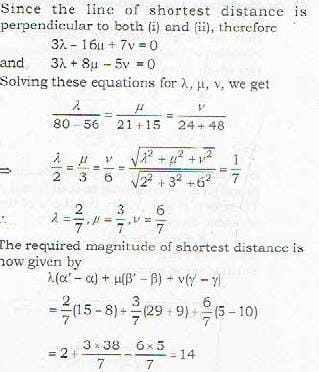Test: Dimensional Geometry - 9 - Question 18

The equation of the line of shortest distance between the lines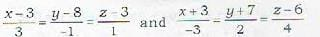is given by

Detailed Solution for Test: Dimensional Geometry - 9 - Question 18

The given lines are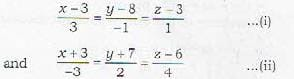Let the line of shortest distance meet the line (i) and (ii) in points P and Q respectively. Then the coordinates of P and Q will be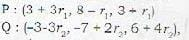where r1, and r2, are such that the line joining P and Q (line of shortest distance) is perpendicular to both (i) and (ii).
The direction ratios of the line PQ are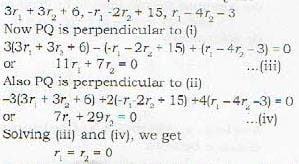The coordinates of points P and Q ,so that PQ becomes the line of shortest distance are (3, 8, 3) and (-3, -7, 6) respectively.
The direction ratios of PQ are
6,15,-3 or 2,5,-1
Required equations of the line PQ of shortest distance are given by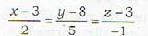Test: Dimensional Geometry - 9 - Question 19

Which is a wrong statement? The three planes
a1x + b1y + c1z + d1 = 0
a2x + b2y + c2z + d2 = 0
a3x + b3y + c3z + d3 = 0
have a common line of intersection, if

Detailed Solution for Test: Dimensional Geometry - 9 - Question 19

The three planes are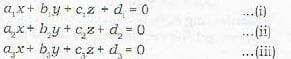Denote the determinants as follows: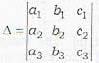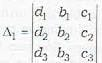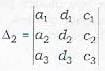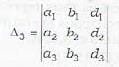Note that Δ is th e coefficients of x, y and z . Δ1, Δ2 and Δ3, are obtained from Δ by replacing its first column, 2nd column and 3rd column by d1, d2 and d3, respectively.
Also note that if the three planes are to intersect in a line, then no two of them are parallel. Under this case, the line of intersection of any two planes will lie on the third plane. Now the line of intersection of (i) and (ii) is given by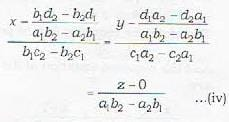Since line (iv) lies on plane (iii), therefore is perpendicuar to the normal to the plane (iii)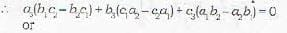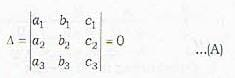Also since line (iv) lies on plane (iii), therefore the coordinates of any point on the line will satisfy the equation of plane (iii), since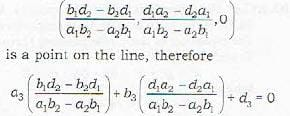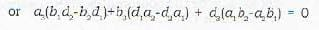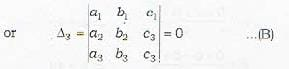Thus (A) and (R) are the required conditions for the three planes to have a common line of intersection.
In a similar way, it can he shown that Δ = 0 and Δ1 = 0 or Δ = 0 and Δ2 = 0 are also the required conditions.
∴ (b), (c), (d) are correct.
Remark: To prove that three planes intersect in a line, verify that Δ = 0 and any one: of Δ12 and Δ3 becomes zero.

Test: Dimensional Geometry - 9 - Question 20

Consider the three planes represented by
a1x + b1y + c1z + d1 = 0
a2x + b2y + c2z + d2 = 0
a3x + b3y + c3z + d3 = 0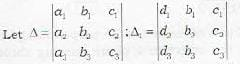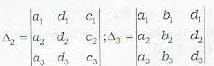then the three planes will form a triangular prism if

## Topic-wise Tests & Solved Examples for IIT JAM Mathematics

27 docs|150 tests
 Use Code STAYHOME200 and get INR 200 additional OFF Use Coupon Code
Information about Test: Dimensional Geometry - 9 Page
In this test you can find the Exam questions for Test: Dimensional Geometry - 9 solved & explained in the simplest way possible. Besides giving Questions and answers for Test: Dimensional Geometry - 9, EduRev gives you an ample number of Online tests for practice

## Topic-wise Tests & Solved Examples for IIT JAM Mathematics

27 docs|150 tests